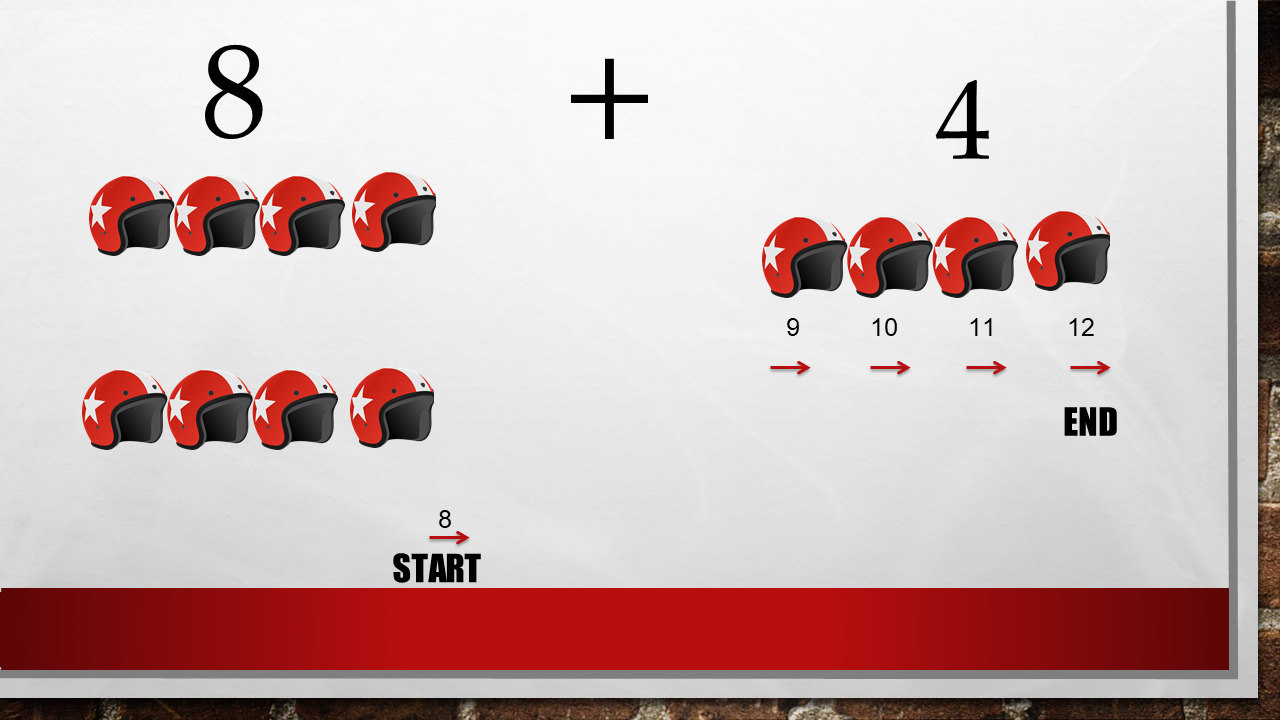1
visibility

Find the sum of the given numbers: 4 + 8

• A

12

• B

11

• C

13

• D

14

The question is exactly 8 + 4 = ?

Now, we can count from any number. Count on!

8 + 4 = ?

We count  4 numbers from 8.

From 8, 9, 10, 11, 12

So: 4 + 8 = 12